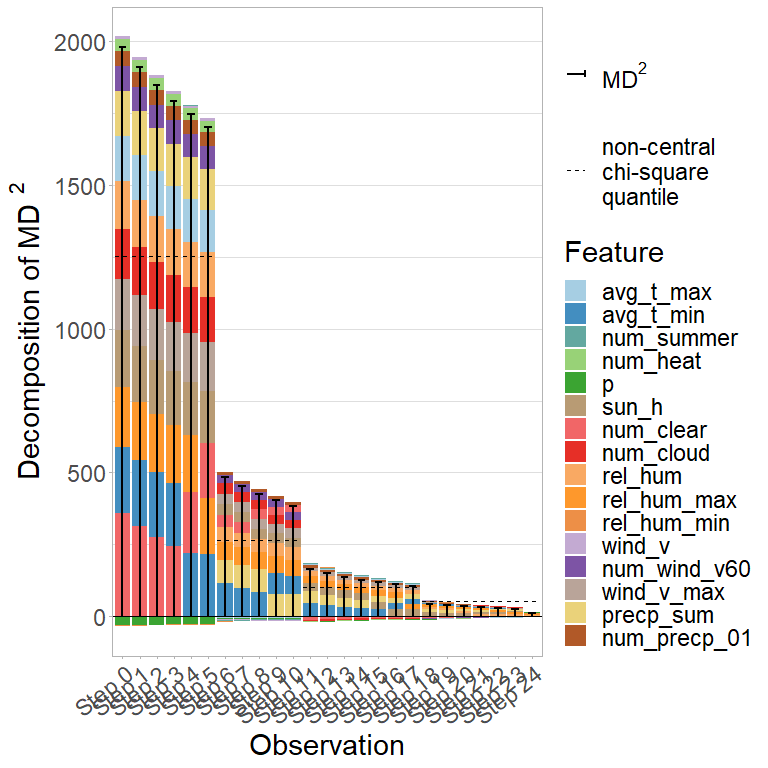# ShapleyOutlier examples

In this file, we present two examples based on real-world data to illustrate the use of the functions from the ShapleyOutlier package.

library(ShapleyOutlier)
library(robustHD)
library(dplyr)
#>
#> Attache Paket: 'dplyr'
#> Die folgenden Objekte sind maskiert von 'package:stats':
#>
#>     filter, lag
#> Die folgenden Objekte sind maskiert von 'package:base':
#>
#>     intersect, setdiff, setequal, union
library(tidyr)
# library(tidyverse)
library(cellWise)

# TopGear dataset

First, we analyze the TopGear dataset from the robustHD package. We dplyr::select all numeric variables except the verdict variable and use a logarithmic transformation for five variables to obtain more symmetrical marginal distributions. We robustly center and scale each column using the median and the MAD and estimate the covariance using the MCD estimator.

data(TopGear)

rownames(TopGear) = paste(TopGear[,1],TopGear[,2])
myTopGear <- TopGear[,-31] #removing the verdict variable
myTopGear <- myTopGear[,sapply(myTopGear,function(x)any(is.numeric(x)))]
myTopGear <- myTopGear[!apply(myTopGear,1, function(x)any(is.na(x))),]
myTopGear <- myTopGear[,-2]
# Transform some variables to get roughly gaussianity in the center:
transTG = myTopGear
transTG$Price = log(myTopGear$Price)
transTG$Displacement = log(myTopGear$Displacement)
transTG$BHP = log(myTopGear$BHP)
transTG$Torque = log(myTopGear$Torque)
transTG$TopSpeed = log(myTopGear$TopSpeed)

transTG <- transTG %>% rename("log(Price)" = Price,
"log(Displacement)" = Displacement,
"log(BHP)" = BHP,
"log(Torque)" = Torque,
"log(TopSpeed)" = TopSpeed)

X <- as.matrix(transTG)
X <- robStandardize(X)
n <- nrow(X)
p <- ncol(X)
set.seed(1)
MCD <- covMcd(X, nsamp = "best")
#> Warning in .fastmcd(x, h, nsamp, nmini, kmini, trace = as.integer(trace)): 'nsamp = "best"' allows maximally 100000 subsets;
#> computing these subsets of size 12 out of 245
mu <-MCD$center Sigma <- MCD$cov
Sigma_inv <- solve(MCD$cov) phi <- shapley(x = X, mu = mu, Sigma = Sigma_inv, inverted = TRUE)$phi
colnames(phi) <- colnames(transTG)
rownames(phi) <- rownames(transTG)
md <- rowSums(phi)
chi2.q <- 0.99
crit <- sqrt(qchisq(chi2.q,p))

## Explaining multivariate outlyingness

In the following, we use the SCD and MOE procedure to analyze the TopGear data. We focus on the six observations with the highest Mahalanobis distance.

n_obs <- 6
TopGear_SCD <- SCD(x = X[names(sort(md, decreasing = TRUE)[1:n_obs]),], mu, Sigma, Sigma_inv, step_size = 0.1, min_deviation = 0.2)
plot(TopGear_SCD, type = "bar", md_squared = FALSE)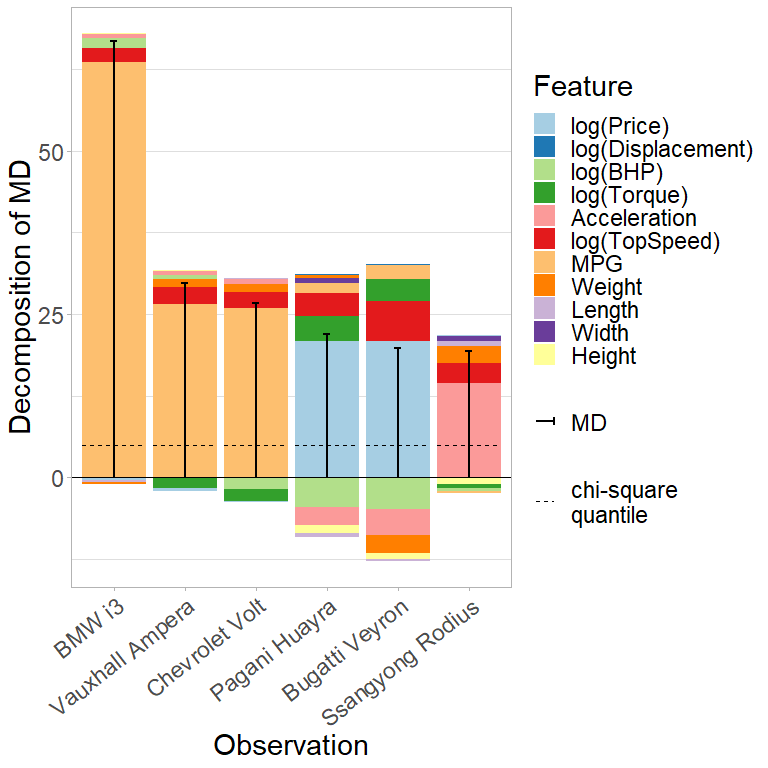TopGear_MOE <- MOE(x = X[names(sort(md, decreasing = TRUE)[1:n_obs]),], mu, Sigma, Sigma_inv, step_size = 0.1, local = TRUE, min_deviation = 0.2)
plot(TopGear_MOE, type = "bar", md_squared = FALSE)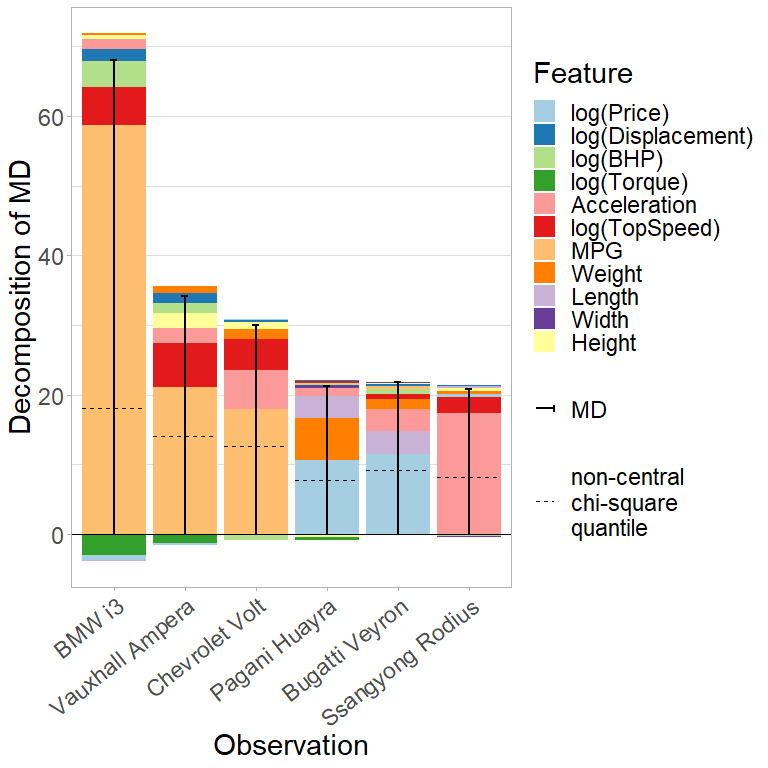As a comparison, we use the cellHandler procedure from the cellWise package and explain the results using Shapley values.

X_sub <- X[names(sort(md, decreasing = TRUE)[1:n_obs]),]
X_sub_cH <- cellHandler(X_sub, mu = mu, Sigma = Sigma)$Ximp explain_cH <- shapley(x = X_sub, mu = mu, Sigma = Sigma_inv, inverted = TRUE, cells = (X_sub != X_sub_cH)) plot(explain_cH, abbrev.var = FALSE, abbrev.obs = FALSE, md_squared = FALSE)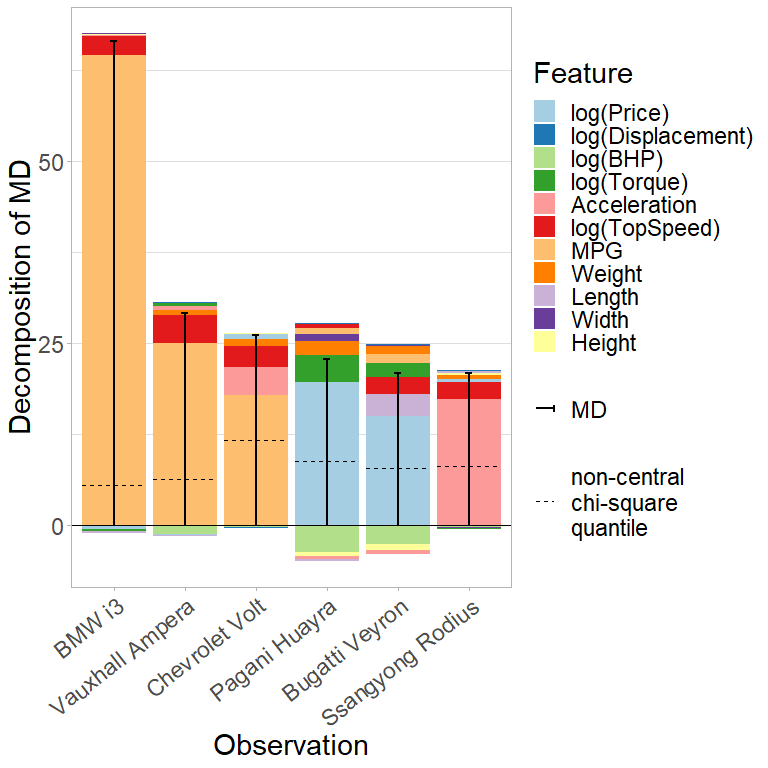## Analyzing cellwise outliers We plot the outlying cells according to the SCD, MOE, and cellHandler procedure. TopGear_SCD_rescaled <- TopGear_SCD TopGear_SCD_rescaled$x_original <- t(apply(TopGear_SCD_rescaled$x_original,1, function(x) x*attr(X, "scale") + attr(X, "center"))) TopGear_SCD_rescaled$x <- t(apply(TopGear_SCD_rescaled$x,1, function(x) x*attr(X, "scale") + attr(X, "center"))) plot(TopGear_SCD_rescaled, type = "cell") + coord_flip() #> Coordinate system already present. Adding new coordinate system, which will #> replace the existing one.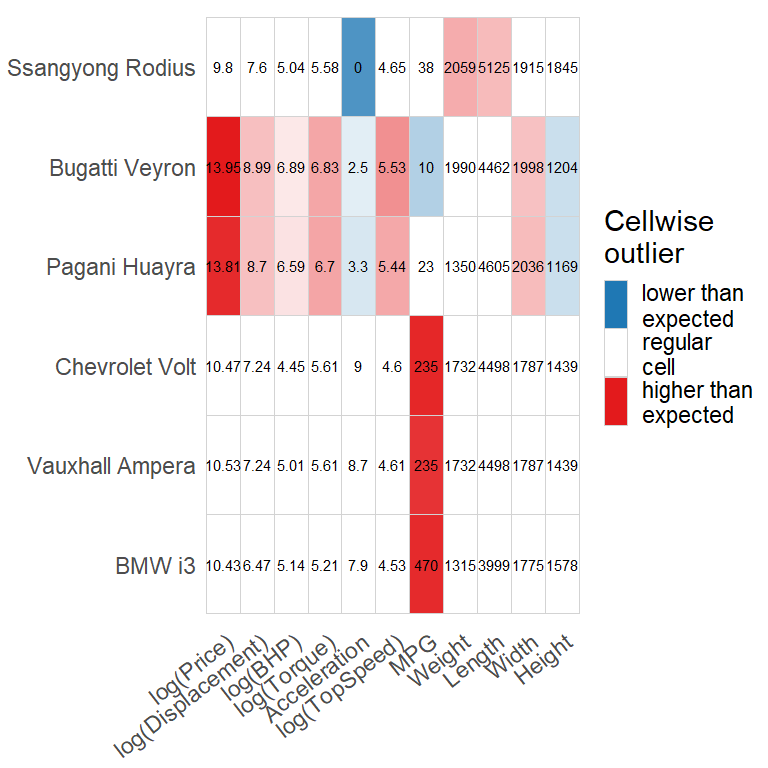TopGear_MOE_rescaled <- TopGear_MOE TopGear_MOE_rescaled$x_original <- t(apply(TopGear_MOE_rescaled$x_original,1, function(x) x*attr(X, "scale") + attr(X, "center"))) TopGear_MOE_rescaled$x <- t(apply(TopGear_MOE_rescaled$x,1, function(x) x*attr(X, "scale") + attr(X, "center"))) plot(TopGear_MOE_rescaled, type = "cell") + coord_flip() #> Coordinate system already present. Adding new coordinate system, which will #> replace the existing one.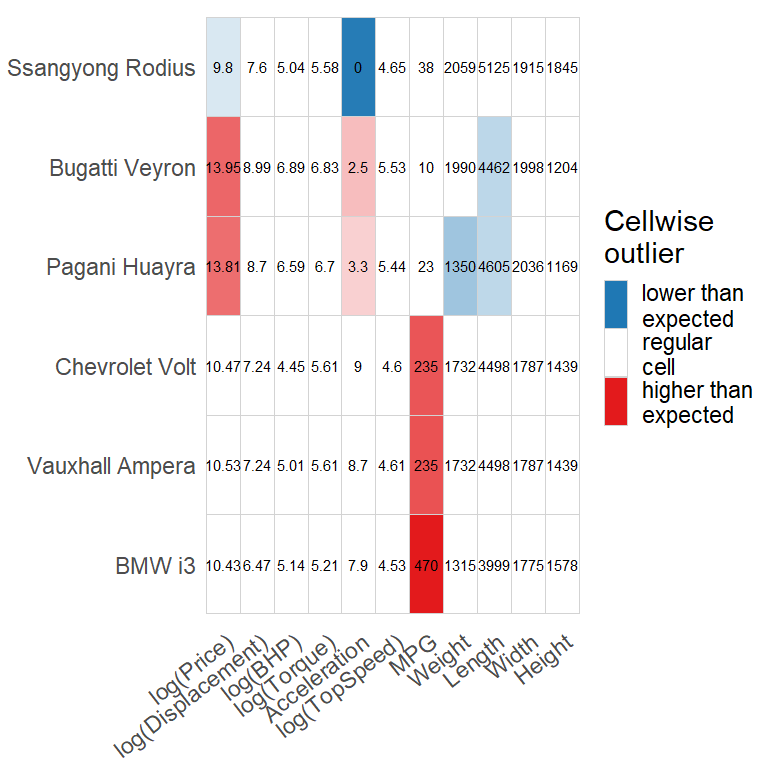plot(x = new_shapley_algorithm(x = t(apply(X_sub_cH,1, function(x) x*attr(X, "scale") + attr(X, "center"))), phi = explain_cH$phi,
x_original =  t(apply(X_sub,1, function(x) x*attr(X, "scale") + attr(X, "center")))),
type = "cell") + coord_flip()
#> replace the existing one.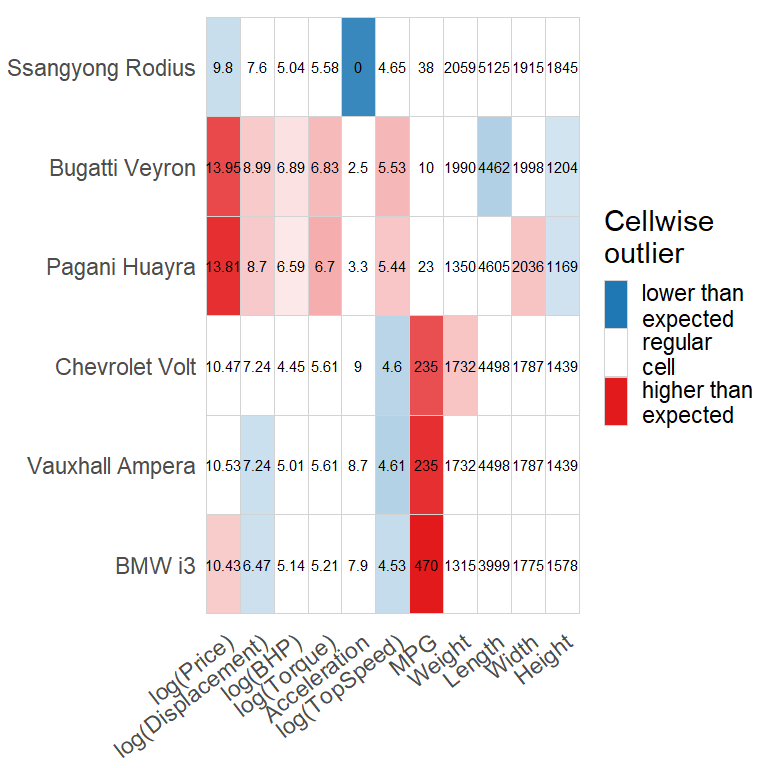## Shapley interaction indices

Comparison of the pairwise outlyingness scores based on the Shapley interaction indices for two cars.

ind <- 3
interaction1 <- shapley_interaction(X[names(sort(md, decreasing = TRUE)[ind]),], TopGear_MOE$mu_tilde[ind,], Sigma) interaction2 <- shapley_interaction(X[names(sort(md, decreasing = TRUE)[ind+1]),], TopGear_MOE$mu_tilde[ind+1,], Sigma)
dimnames(interaction1) <- list(c("Price", "Displ.", "BHP", "Torque", "Acc", "T.Speed", "MPG", "Weight", "Length", "Width", "Height"),
c("Price", "Displ.", "BHP", "Torque", "Acc", "T.Speed", "MPG", "Weight", "Length", "Width", "Height"))
dimnames(interaction2) <- dimnames(interaction1)
plot(interaction1, abbrev = FALSE, title = names(sort(md, decreasing = TRUE)[ind]))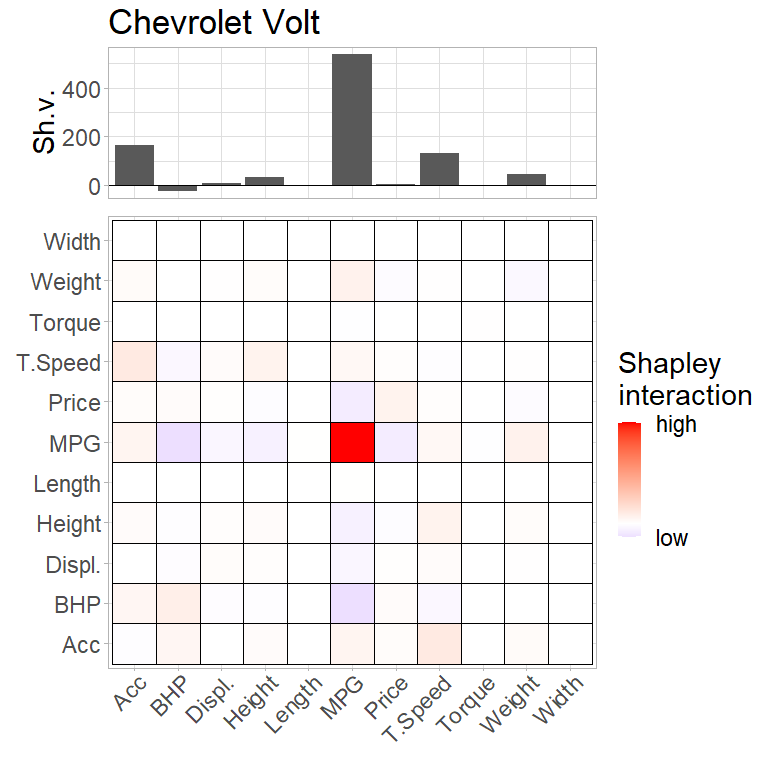plot(interaction2, abbrev = FALSE, title = names(sort(md, decreasing = TRUE)[ind+1]))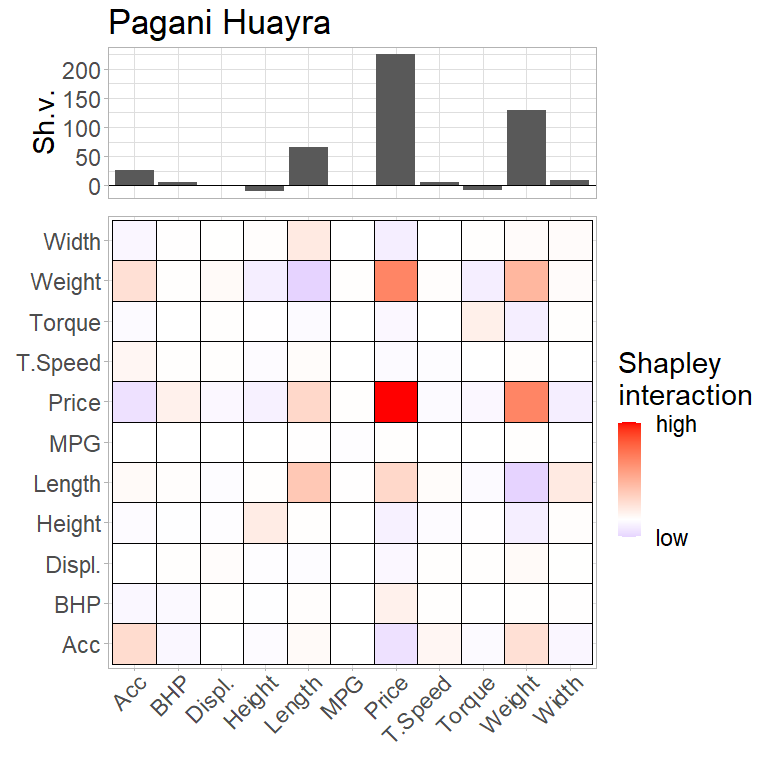# WeatherVienna

In the following, we analyze the monthly data from the weather station Hohe Warte in Vienna. We only consider the years after 1955, exclude some variables to avoid redundancy, and compute the average values of the summer months June, July, and August. The resulting dataset contains information about the average summer weather in Vienna from 1955 to 2022. We robustly center and scale each column using the median and the MAD and estimate the covariance using the MCD estimator.

data("WeatherVienna")

weather_summer <- WeatherVienna %>% dplyr::select(-c(t, t_max, t_min, p_max, p_min)) %>%
drop_na() %>%
filter(month %in% c("JUN", "JUL", "AUG")) %>%
filter(year >= 1955) %>%
group_by(year) %>%
dplyr::select(-month) %>%
summarise(across(.cols = everything(), function(x) mean(x)))

X <- weather_summer %>% dplyr::select(-c(num_frost, num_ice, year))
rownames(X) <- weather_summer$year #> Warning: Setting row names on a tibble is deprecated. X <- robStandardize(X) n <- nrow(X) p <- ncol(X) set.seed(1) MCD <- covMcd(X, alpha = 0.5, nsamp = "best") #> Warning in .fastmcd(x, h, nsamp, nmini, kmini, trace = as.integer(trace)): 'nsamp = "best"' allows maximally 100000 subsets; #> computing these subsets of size 17 out of 68 mu <-MCD$center
Sigma <- MCD$cov Sigma_inv <- solve(MCD$cov)
phi <- shapley(x = X, mu = mu, Sigma = Sigma_inv, inverted = TRUE)$phi colnames(phi) <- colnames(X) rownames(phi) <- rownames(X) md <- rowSums(phi) chi2.q <- 0.99 crit <- sqrt(qchisq(chi2.q,p)) ## Explaining multivariate outlyingness We use the SCD and MOE procedure to analyze the weather data and compare it to the cellHandler procedure. weather_SCD <- SCD(x = X, mu, Sigma, Sigma_inv, step_size = 0.1, min_deviation = 0.2) plot(weather_SCD, abbrev.var = FALSE, abbrev.obs = FALSE, md_squared = FALSE, sort.obs = FALSE, type = "bar")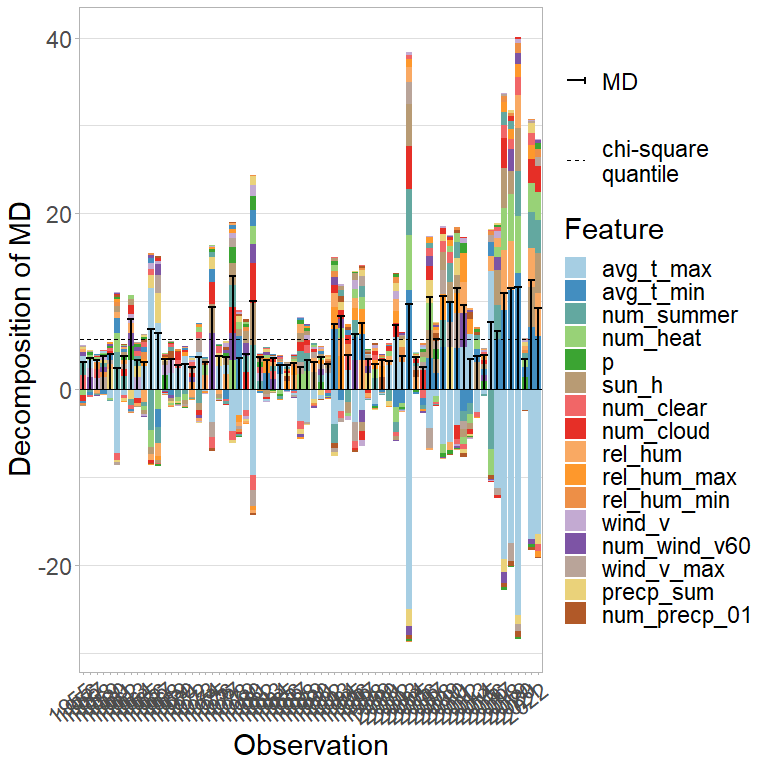weather_MOE <- MOE(x = X, mu, Sigma, Sigma_inv, step_size = 0.1, local = TRUE, min_deviation = 0.2) plot(weather_MOE, abbrev.var = FALSE, abbrev.obs = FALSE, md_squared = FALSE, sort.obs = FALSE, type = "bar")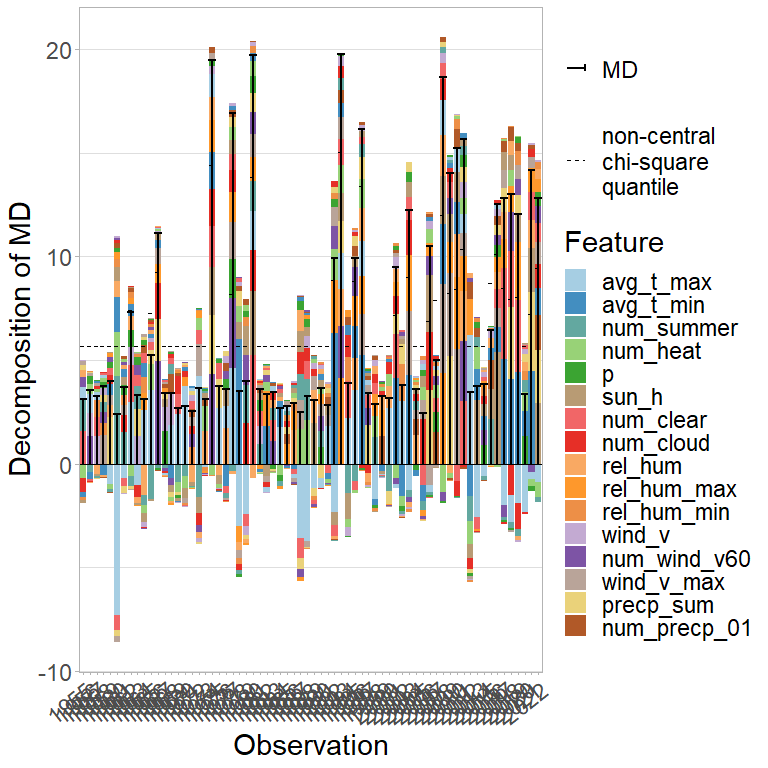X_cH <- cellHandler(as.matrix(X), mu = mu, Sigma = Sigma)$Ximp
explain_cH <- shapley(x = X, mu = mu, Sigma = Sigma_inv, inverted = TRUE, cells = (X != X_cH))
plot(explain_cH, abbrev.var = FALSE, abbrev.obs = FALSE, md_squared = FALSE)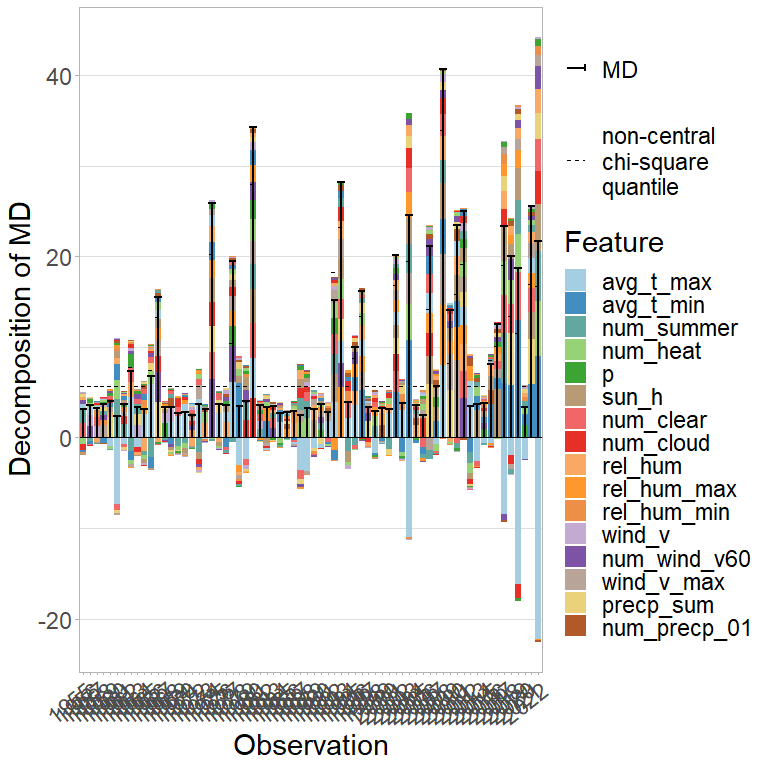## Analyzing cellwise outliers

We plot the outlying cells according to the SCD, MOE, and cellHandler procedure.

weather_SCD_rescaled <- weather_SCD
weather_SCD_rescaled$x_original <- t(apply(weather_SCD_rescaled$x_original,1, function(x) x*attr(X, "scale") + attr(X, "center")))
weather_SCD_rescaled$x <- t(apply(weather_SCD_rescaled$x,1, function(x) x*attr(X, "scale") + attr(X, "center")))
plot(weather_SCD_rescaled, type = "cell", n_digits = 0, continuous_rowname = 10, rotate_x = FALSE)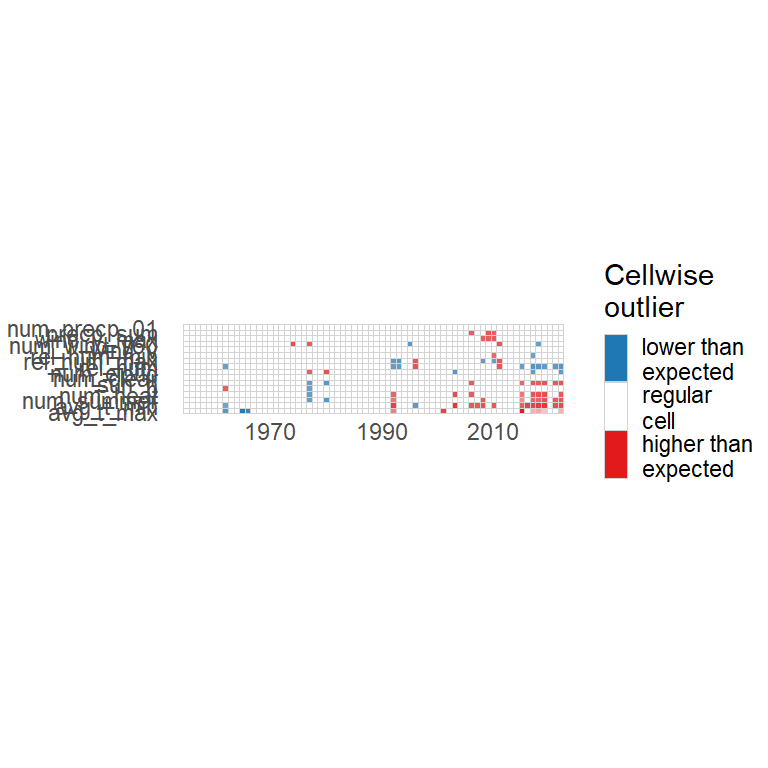weather_MOE_rescaled <- weather_MOE
weather_MOE_rescaled$x_original <- t(apply(weather_MOE_rescaled$x_original,1, function(x) x*attr(X, "scale") + attr(X, "center")))
weather_MOE_rescaled$x <- t(apply(weather_MOE_rescaled$x,1, function(x) x*attr(X, "scale") + attr(X, "center")))
plot(weather_MOE_rescaled, type = "cell", n_digits = 0, continuous_rowname = 10, rotate_x = FALSE)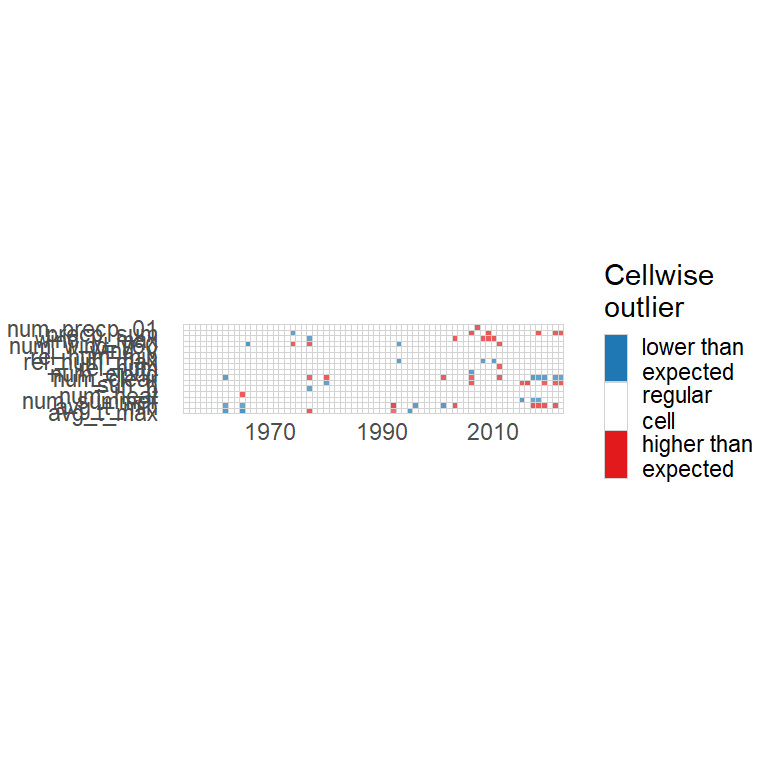plot(x = new_shapley_algorithm(x = t(apply(X_cH,1, function(x) x*attr(X, "scale") + attr(X, "center"))),
phi = explain_cH$phi, x_original = t(apply(X,1, function(x) x*attr(X, "scale") + attr(X, "center")))), type = "cell", n_digits = 0, continuous_rowname = 10, rotate_x = FALSE)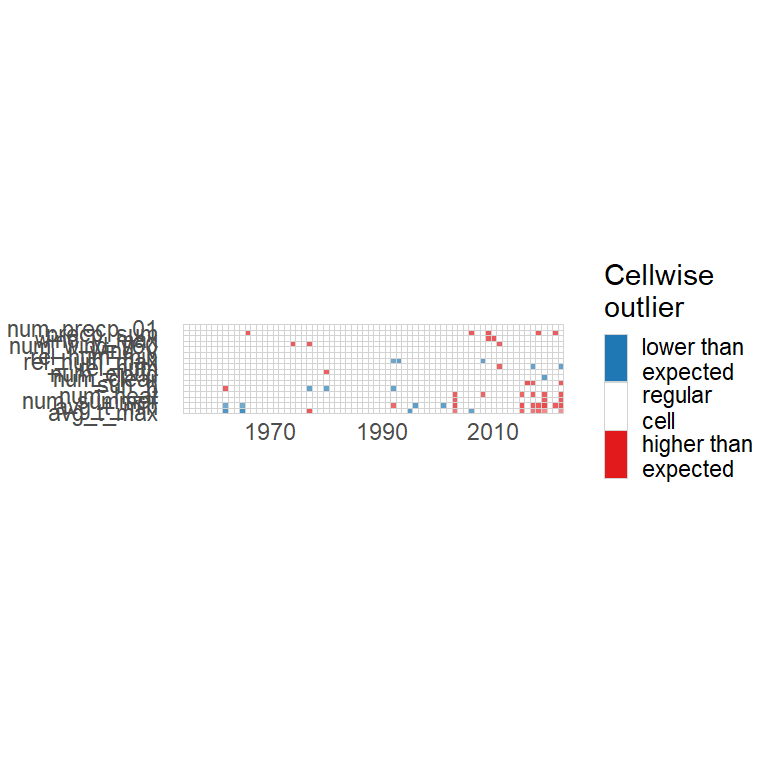## Shapley interaction indices ind <- 67 #year 2021 interaction1 <- shapley_interaction(as.numeric(X[ind,]), mu, Sigma) interaction2 <- shapley_interaction(as.numeric(X[ind,]), weather_MOE$mu_tilde[ind,], Sigma)

plot(interaction1, abbrev = FALSE, legend = FALSE, title = "SCD: year 2021", text_size = 16)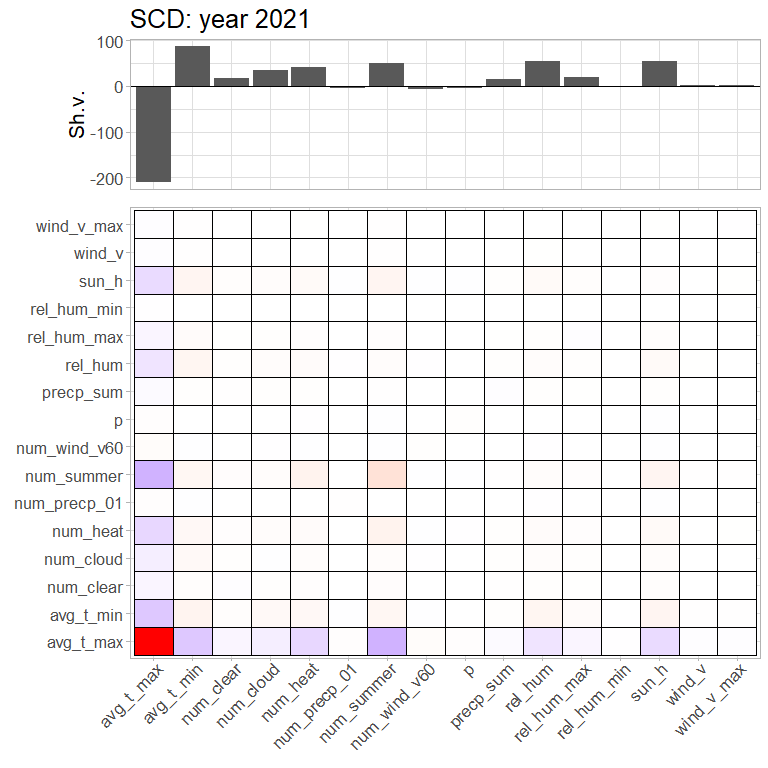plot(interaction2, abbrev = FALSE, title = "MOE: year 2021", text_size = 16)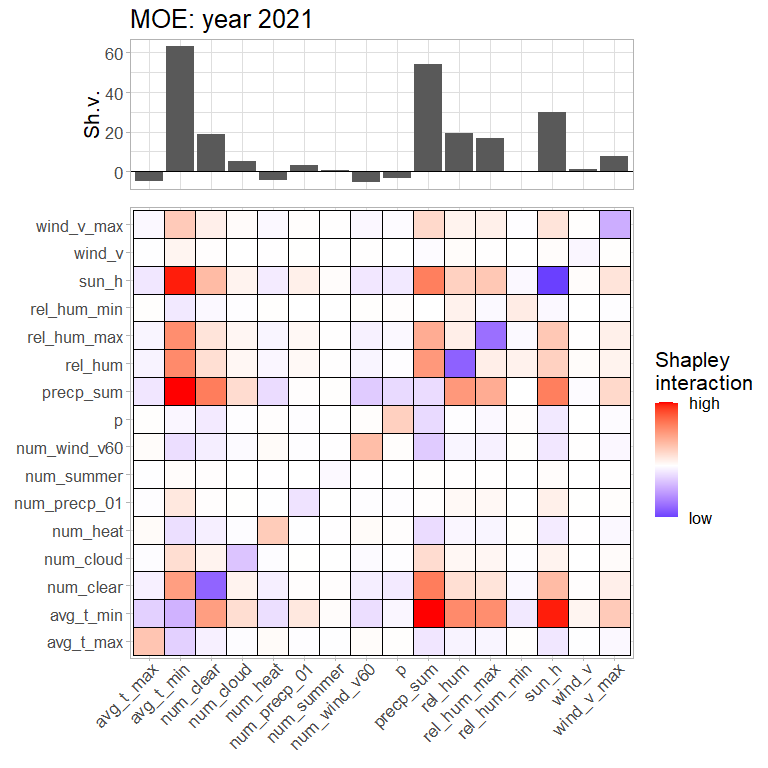## Iterations of SCD and MOE for the year 2021

phi_SCD <- unique(weather_SCD$phi_history[[ind]]) rownames(phi_SCD) <- paste("Step", 0:(nrow(phi_SCD)-1)) plot(new_shapley(phi = phi_SCD), abbrev.var = FALSE, abbrev.obs = FALSE, sort.obs = FALSE, sort.var = FALSE)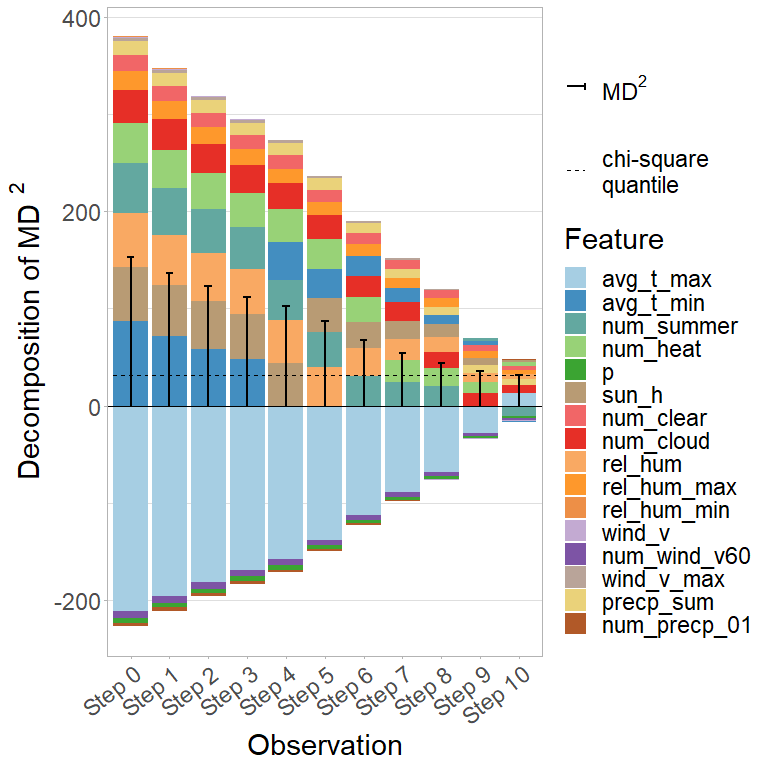phi_MOE <- weather_MOE$phi_history[[ind]]
mu_tilde_MOE <- weather_MOE\$mu_tilde_history[[ind]]
non_centrality_MOE <- apply(mu_tilde_MOE, 1, function(x) mahalanobis(x, mu, Sigma_inv, inverted = TRUE))
rownames(phi_MOE) <- paste("Step", 0:(nrow(phi_MOE)-1))
plot(new_shapley(phi = phi_MOE,
mu_tilde = mu_tilde_MOE,
non_centrality = non_centrality_MOE),
abbrev.var = FALSE, abbrev.obs = FALSE, sort.obs = FALSE, sort.var = FALSE)